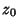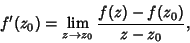## Differentiable

A Function is said to be differentiable at a point if its Derivative exists at that point. Letandon some regioncontaining the point. Ifsatisfies the Cauchy-Riemann Equations and has continuous first Partial Derivatives at, thenexists and is given byand the function is said to be Complex Differentiable. Amazingly, there exist Continuous Functions which are nowhere differentiable. Two examples are the Blancmange Function and Weierstraß Function.

See also Blancmange Function, Cauchy-Riemann Equations, Complex Differentiable, Continuous Function, Derivative, Partial Derivative, Weierstraß Function